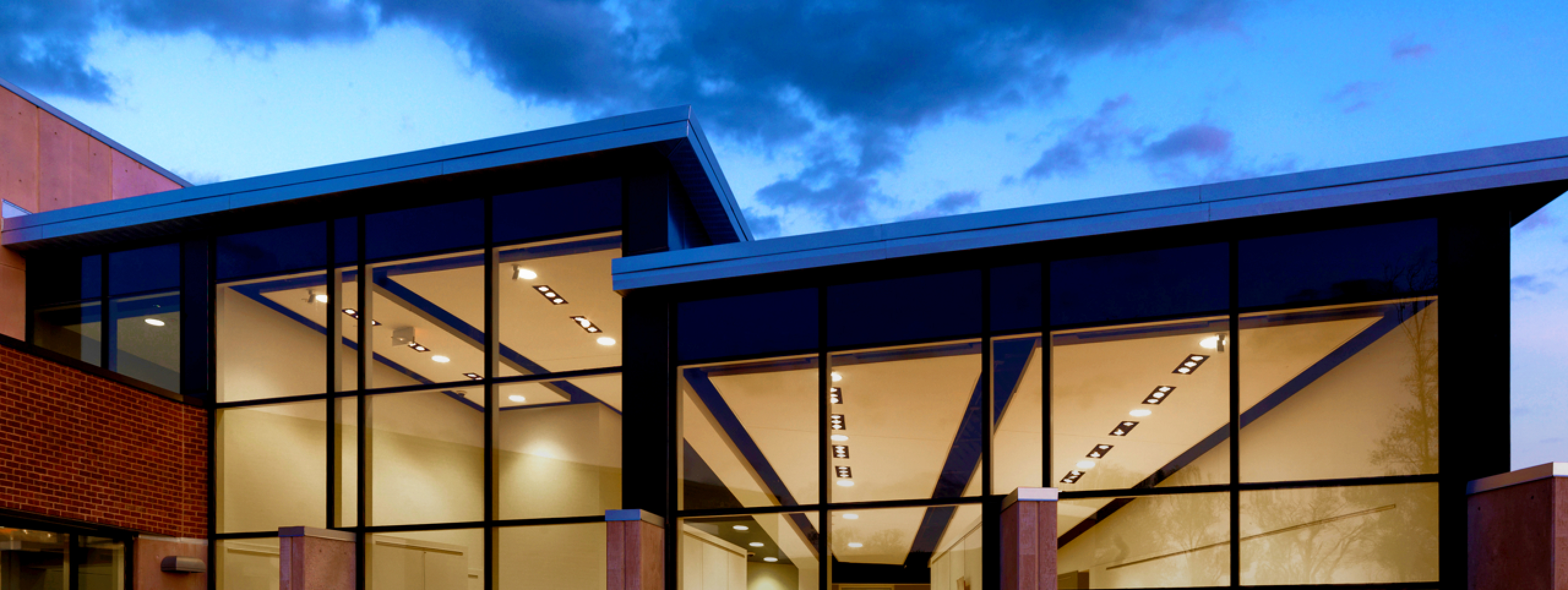# Calculus and Analytical Geometry II

## Course Overview

Course Code: MATH-267

Department: Mathematics

Credit Hours: 4.00

Illinois Articulation Initiative Number: IAI MTH 902

Textbook Information: Bookstore website

Topics include (but are not limited to) the following: limits and continuity; definition of derivative: rate of change, slope; derivatives of polynomial and rational functions; the chain rule; implicit differentials; approximation by differentials; higher order derivatives; Rolle's Theorem: mean value theorem; applications of the derivative; anti-derivative; the definite integral; the fundamental theorem of calculus; area, volume, other applications of the integral; the calculus of the trigonometric functions; logarithmic and exponential functions; techniques of integration, including numerical methods; indeterminate forms: L'Hopital's rule; improper integrals; sequences and series, convergence tests, Taylor series; functions of more than one variable, partial derivatives; the differential, directional derivatives, gradients; double and triple integrals: evaluation and applications. Prerequisite: Completion of MATH 166 with a grade of C or better.

## Course Schedule

Filters:

Term: 2021SP

Section Name: MATH-267-MC01D

Location: Main Campus

Available Seats: 9

Faculty Name: D. Watkins

Min Credits: 4.00